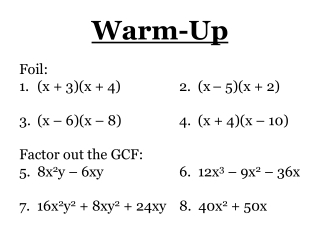Download PresentationWarm-Up

# Warm-Up

Download Presentation## Warm-Up

- - - - - - - - - - - - - - - - - - - - - - - - - - - E N D - - - - - - - - - - - - - - - - - - - - - - - - - - -
##### Presentation Transcript

1. Warm-Up Foil: (x + 3)(x + 4) 2. (x– 5)(x + 2) (x – 6)(x – 8) 4. (x + 4)(x – 10) Factor out the GCF: 8x2y – 6xy 6. 12x3 – 9x2 – 36x 7. 16x2y2 + 8xy2 + 24xy 8. 40x2 + 50x

2. Section 8-5 Add and Subtract Rational Expressions

3. Example 1 Perform the indicated operation: a.) 12 2 5x 5x b.) 3x 1 2x + 5 2x + 5 = 10 5x – = 2 x = 3x + 1 2x + 5 +

4. Example 2 Find the least common multiple of 5x2 – 45 4x2 + 24x+ 36 Step 1:Factor each polynomial. 5(x2 – 9) 5(x – 3)(x + 3) Step 2:Form the LCM by writing each factor to the highest power it occurs in either polynomial. 4(x2 + 6x + 9) 4(x + 3)(x + 3) 20(x – 3)(x + 3)2

5. Example 3 Add: 3 2x 10x2 5x2 – 10x + Step 1:Factor the denominator. 3 10x2 2x 5x(x –2) + Step 2:LCM is 10x2(x – 2). 3 10x2 (x – 2) (x – 2) 2x 5x(x –2) 2x 2x • + • 3x – 6 10x2 (x – 2) Step 3:Multiply. 4x2 10x2 (x –2) + Step 4:Add numerators. 4x2 + 3x – 6 10x2 (x – 2)

6. Example 4 Simplify: 6 1 x – 5 x 3 2 x x – 5 Step 1:Find LCM of all denominators. + x(x – 5) x(x – 5) • – x(x – 5) 6x + x – 5 7x – 5 x – 15 Step 2:Multiply by the LCM. = 3(x – 5) – 2x Step 3:Simplify.

7. Homework Section 8-5 Pages 586 –587 1, 3, 5, 6, 10, 12, 13, 17, 18, 20, 21, 22, 27, 30, 31, 32, 35